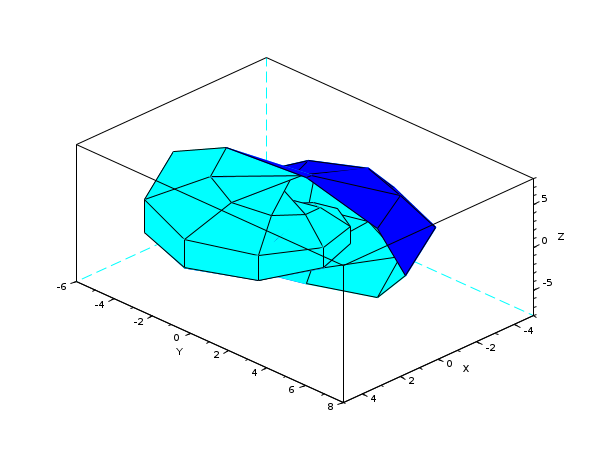Scilab Home page | Wiki | Bug tracker | Forge | Mailing list archives | ATOMS | File exchange
Change language to: English - Français - Português - 日本語 -

See the recommended documentation of this function

Справка Scilab >> Графики > 3d_plot > eval3dp

# eval3dp

compute facets of a 3D parametric surface

### Calling Sequence

`[Xf,Yf,Zf]=eval3dp(fun,p1,p2)`

### Arguments

Xf,Yf,Zf

matrices of size (4,n-1*m-1). `Xf(:,i)` ,`Yf(:,i)` and `Zf(:,i)` are respectively the x-axis, y-axis and z-axis coordinates of the 4 points of the ith four sided facet.

fun

a Scilab function.

p1

a vector of size `n`.

p2

a vector of size `m`.

### Description

`eval3dp` computes a four sided facets representation of a 3D parametric surface defined by the function `fun`. `fun(p1,p2)` computes the x-axis ,y-axis and z-axis coordinates of the corresponding points on the surface, as [x(i),y(i),z(i)]=fun(p1(i),p2(i)). This is used for efficiency.

### Examples

```p1=linspace(0,2*%pi,10);
p2=linspace(0,2*%pi,10);
deff("[x,y,z]=scp(p1,p2)",["x=p1.*sin(p1).*cos(p2)";..
"y=p1.*cos(p1).*cos(p2)";..
"z=p1.*sin(p2)"])
[Xf,Yf,Zf]=eval3dp(scp,p1,p2);
plot3d(Xf,Yf,Zf)```